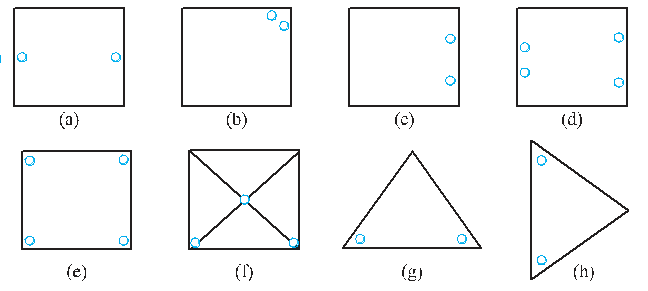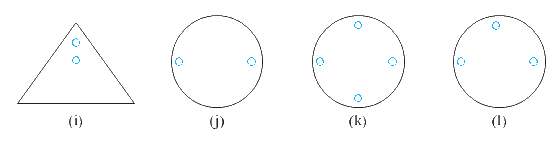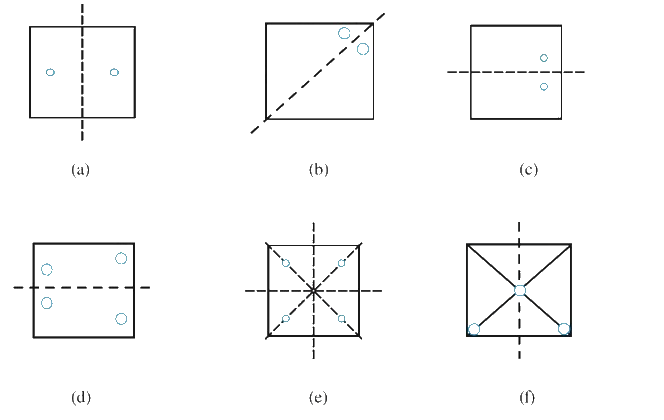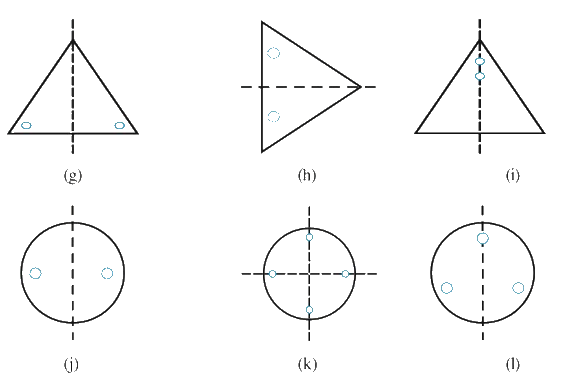# Ex.14.1 Q1 Symmetry - NCERT Maths Class 7

## Question

Copy the figures with punched holes and find the axes of symmetry for the following:Video Solution
Symmetry
Ex 14.1 | Question 1

## Text Solution

What is known?

The figure with punched holes.

What is unknown?

The axis of symmetry.

Reasoning:

This question is very simple. You have to draw different lines and check which line will divide it into exactly same parts, that line will be called the line of symmetry.

Steps:(a) It has one line of symmetry.

(b) It has one line of symmetry.

(c) It has one line of symmetry.

(d) It has one line of symmetry.

(e) It has four lines of symmetry.

(f) It has one line of symmetry.

(g) It has one line of symmetry.

(h) It has one line of symmetry.

(i) It has one line of symmetry.

(j) It has one line of symmetry.

(k) It has two lines of symmetry.

(l) It has one line of symmetry.

Learn from the best math teachers and top your exams

• Live one on one classroom and doubt clearing
• Practice worksheets in and after class for conceptual clarity
• Personalized curriculum to keep up with school